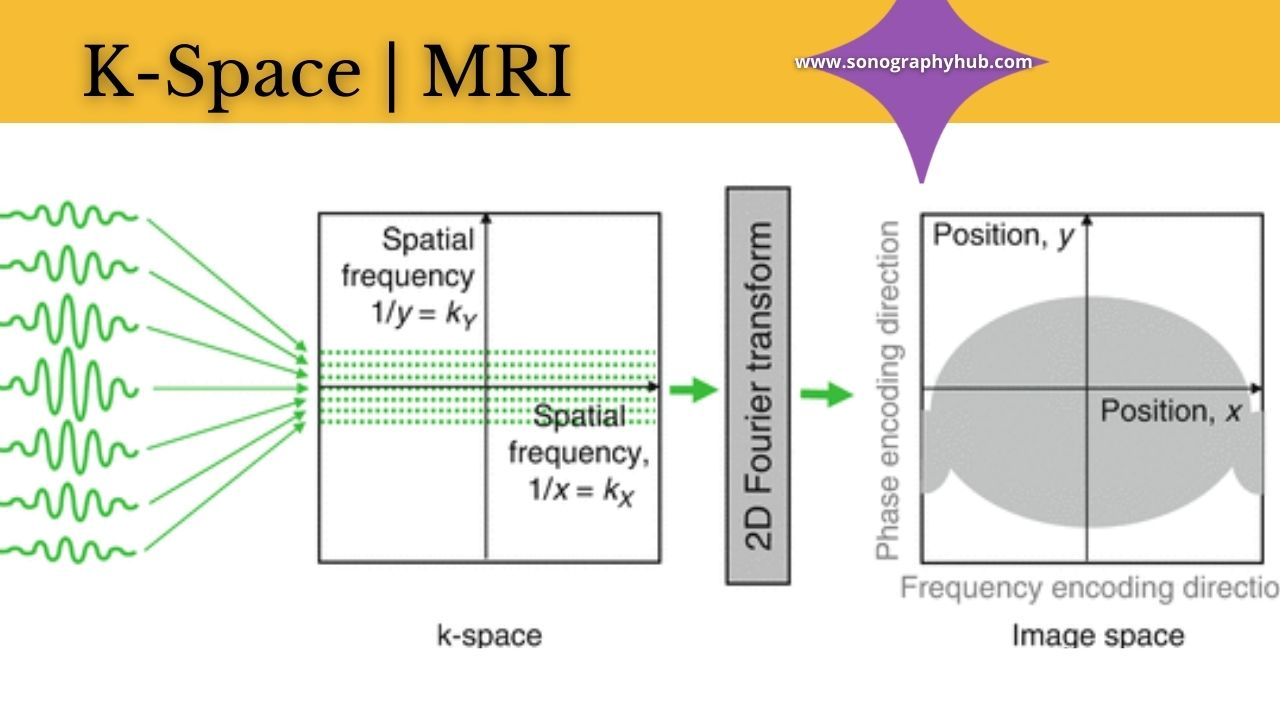# K-Space and Scanning Parameters | MRI

In MRI K-Space is an imaginary space that represents a raw data matrix. It represents the stage between the reception of signals and image formation. After the acquisition, all signals are stored in K-Space in a particular fashion. This raw data from K-Space is used to reconstruct images by Fourier Transformation.K-Space

MRI K-Space has two axes. The horizontal axis represents the phase axis and is centered in the middle of several horizontal lines. The frequency axis of K-Space is vertical and is perpendicular to the phase axis.

Signals are filled in K-space as horizontal lines. The number of MRI K-Space that is filled is determined by the number of different phase encoding steps. If 128 different phase encoding steps are selected then 128 lines of K-space are filled to form an image. In conventional spin-echo imaging one line of K-space is filled to form an image.

An array of signals as lines in the K-Space does not correspond with rows or columns of a pixel in the image. It is a complex transformation of this data into an image where the center part of K-Space represents the contrast of the image and the periphery corresponds with resolution and fine details.

Two halves of K-Space, right and left or upper and lower are exact symmetric conjugate and represent the same information. This fact is used to manipulate K-Space sampling (partial sampling) to get the same image in less time. In fast imaging like signal-shot fast spin-echo sequence such as HASTE, just more than half of K-space is filled. In ultrafast Echo Planar Imaging (EPI)  all the K-Space lines required to form an image are filled within a single TR, thus reducing the scanning time in seconds.

## Major 5 parameters of scanning in MRI K-Space

1. Matrix
2. FOV (field of view)
3. NEX | NSA
4. Flip angle
5. Bandwidth

### 1. Matrix

A pixel (picture element) is the smallest unit of a digital image. The matrix consists of rows and columns of pixels. Matrix of 256 x 256 means the image 256 rows and 256 columns of pixels. The greater the size of the matrix better is the resolution. If the matrix is increased keeping the FOV the same, pixel size will reduce. So smaller the pixel better the resolution. An image acquired with a matrix of 512 x 512 will have better resolution than the image acquired with a matrix of 256 x 256. However, increasing the matrix size increases the scanning time and reduces SNR.

A pixel that has two dimensions is added the smallest unit of the imaging data is called voxel (volume element). Voxel volume is determined by matrix size, FOV, and slice thickness.

### 2. FOV (field of view)

It is the area from which pieces of information are obtained and represented on the image. It is selected as per the requirement of the under examination by the technologist. It should be sufficient enough to cover the area of interest. Increasing FOV imposes SNR but reduces resolutions.

### 3. Number of excitations (NEX) / Number of signals averages (NSA)

It represents the number of times the data is acquired to form the same image. More the number of averages better will be SNR but it increases the scan time.

### 4. Flip angle

It is the angle by which the longitudinal magnetization vector is rotated away from Z-axis by an RF pulse. Shorter flip angles are used in Gradient-Echo (GRE) sequences. The flip angle also determines the contrast in GRE sequences. T1-weighting of a GRE sequence increases with increasing flip angle. Flip angles are specific to the sequence and are not usually changed at the time of scanning.

### 5. Bandwidth

Bandwidth is the range of frequencies. Reducing receiver bandwidth of the receiver RF coil increases SNR. However, it also increases chemical shift and minimum TE that can be used.

## Image quality determinants in MRI K-Space

(a) Signal-to-noise ratio (SNR) is the ratio of a useful signal to an unwanted signal (noise) in the image. It is one of the most important image quality determinants. An image with high SNR is less grainy and has more details. Factors affecting SNR could be fixed or changeable.

Fixed factors include magnetic field strength, pulse sequence design, and tissue characteristics. Factors that can be changed include RF coil, voxel size, NSA, receiver bandwidth, and slice cross talk. SNR improves with a number of averages, FOV, surface, and phased array coils.

(b) Spatial resolution is the ability to discern two points as distinct and separate. Smaller the points that are seen separately better is the resolution. It improves with matrix size.

(c) Contrast is an image is the signal intensity difference between adjacent structures. More the contrast easier will be a determination of the adjacent structures. The contrast in an image is determined by tissue-specific parameters, such as T1, T2, proton density, chemical environment, and macromolecules, and hydration layers. The Contrast-to-noise ratio (CNR) is the difference in SNR between adjacent structures.

### Imaging time

aT = TR x N* x NEX

Where:
aT = acquisition time
TR = Time to repeat
N* = Number of pixels (matrix)
NEX = Number of excitations

## When you scan...

While scanning, the aim should be to get good quality images with reasonable SNR and spatial resolution in a shorter time.